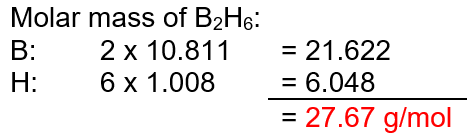# Problem: Compounds of boron and hydrogen are remarkable for their unusual bonding and also for their reactivity. With the more reactive halogens, for example, diborane (B2H6) forms trihalides even at low temperatures:B2H6 (g) + 6Cl2 (g) ⟶ 2BCl3 (g) + 6HCl (g)       ΔH = −755.4 kJWhat is ΔH per kilogram of diborane that reacts?

###### FREE Expert Solution

Enthalpy, ΔH, is an extensive property and state function that represents the total amount of heat within a chemical reaction or system

In the given chemical reaction, the enthalpy is -755.4 kJ per mol of the reaction.

1 mol of the reaction uses 1 mol of B2H(diborane)

Moles can be related to mass using molar mass.94% (212 ratings)###### Problem Details

Compounds of boron and hydrogen are remarkable for their unusual bonding and also for their reactivity. With the more reactive halogens, for example, diborane (B2H6) forms trihalides even at low temperatures:

B2H6 (g) + 6Cl2 (g) ⟶ 2BCl3 (g) + 6HCl (g)       ΔH = −755.4 kJ

What is ΔH per kilogram of diborane that reacts?

Frequently Asked Questions

What scientific concept do you need to know in order to solve this problem?

Our tutors have indicated that to solve this problem you will need to apply the Thermochemical Equations concept. You can view video lessons to learn Thermochemical Equations. Or if you need more Thermochemical Equations practice, you can also practice Thermochemical Equations practice problems.

What professor is this problem relevant for?

Based on our data, we think this problem is relevant for Professor Mamiya's class at TXSTATE.

What textbook is this problem found in?

Our data indicates that this problem or a close variation was asked in Chemistry: The Molecular Nature of Matter and Change - Silberberg 8th Edition. You can also practice Chemistry: The Molecular Nature of Matter and Change - Silberberg 8th Edition practice problems.